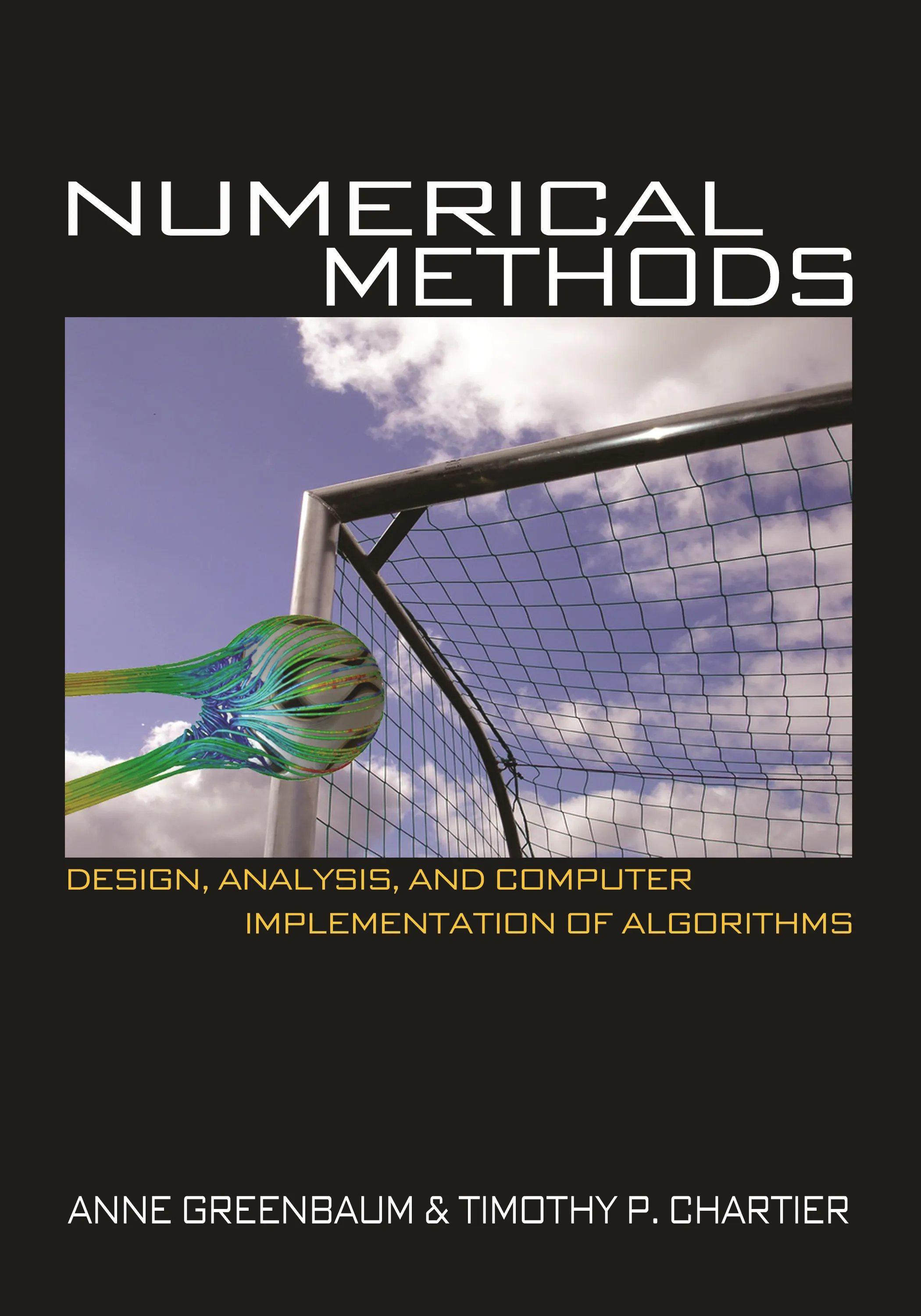#### Numerical Methods

Anne Greenbaum

##### ebooks

Many of our ebooks are available for purchase from these online vendors:

Many of our ebooks are available through library electronic resources including these platforms:

# Numerical Methods: Design, Analysis, and Computer Implementation of Algorithms

A rigorous and comprehensive introduction to numerical analysis## Hardcover

Price:
\$115.00 / £90.00
ISBN:
Published:
Apr 1, 2012
2012
Pages:
464
Size:
7 x 10 in.
Illus:
78 halftones. 145 line illus.

Numerical Methods provides a clear and concise exploration of standard numerical analysis topics, as well as nontraditional ones, including mathematical modeling, Monte Carlo methods, Markov chains, and fractals. Filled with appealing examples that will motivate students, the textbook considers modern application areas, such as information retrieval and animation, and classical topics from physics and engineering. Exercises use MATLAB and promote understanding of computational results.

The book gives instructors the flexibility to emphasize different aspects—design, analysis, or computer implementation—of numerical algorithms, depending on the background and interests of students. Designed for upper-division undergraduates in mathematics or computer science classes, the textbook assumes that students have prior knowledge of linear algebra and calculus, although these topics are reviewed in the text. Short discussions of the history of numerical methods are interspersed throughout the chapters. The book also includes polynomial interpolation at Chebyshev points, use of the MATLAB package Chebfun, and a section on the fast Fourier transform. Supplementary materials are available online.

• Clear and concise exposition of standard numerical analysis topics

• Explores nontraditional topics, such as mathematical modeling and Monte Carlo methods

• Covers modern applications, including information retrieval and animation, and classical applications from physics and engineering

• Promotes understanding of computational results through MATLAB exercises

• Provides flexibility so instructors can emphasize mathematical or applied/computational aspects of numerical methods or a combination

• Includes recent results on polynomial interpolation at Chebyshev points and use of the MATLAB package Chebfun

• Short discussions of the history of numerical methods interspersed throughout

• Supplementary materials available online